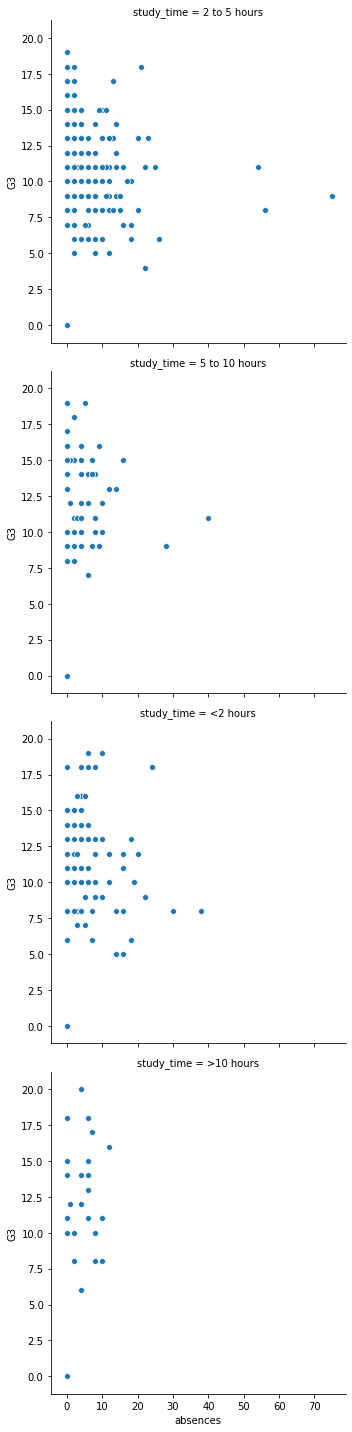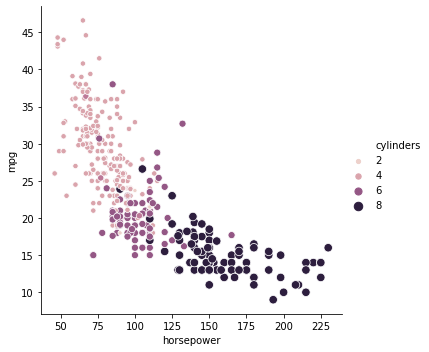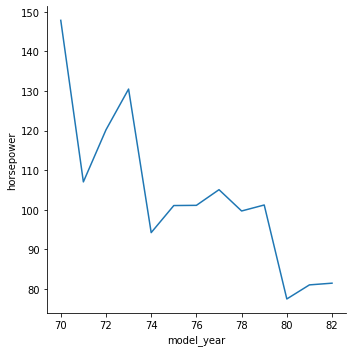import pandas as pd
import matplotlib.pyplot as plt
import seaborn as sns

plt.rcParams['figure.figsize'] = (10, 5)


## Introduction to relational plots and subplots

• Relational plots
• Height vs. Weight
• Number of school absences vs. final grade
• GDP vs. percentile literate

### Creating subplots with col and row

We've seen in prior exercises that students with more absences ("absences") tend to have lower final grades ("G3"). Does this relationship hold regardless of how much time students study each week?

To answer this, we'll look at the relationship between the number of absences that a student has in school and their final grade in the course, creating separate subplots based on each student's weekly study time ("study_time").

student_data = pd.read_csv('./dataset/student-alcohol-consumption.csv', index_col=0)

school sex age famsize Pstatus Medu Fedu traveltime failures schoolsup ... goout Dalc Walc health absences G1 G2 G3 location study_time
0 GP F 18 GT3 A 4 4 2 0 yes ... 4 1 1 3 6 5 6 6 Urban 2 to 5 hours
1 GP F 17 GT3 T 1 1 1 0 no ... 3 1 1 3 4 5 5 6 Urban 2 to 5 hours
2 GP F 15 LE3 T 1 1 1 3 yes ... 2 2 3 3 10 7 8 10 Urban 2 to 5 hours
3 GP F 15 GT3 T 4 2 1 0 no ... 2 1 1 5 2 15 14 15 Urban 5 to 10 hours
4 GP F 16 GT3 T 3 3 1 0 no ... 2 1 2 5 4 6 10 10 Urban 2 to 5 hours

5 rows × 29 columns

sns.relplot(x="absences", y="G3", data=student_data, kind='scatter');sns.relplot(x="absences", y="G3",
data=student_data,
kind="scatter",
col='study_time');sns.relplot(x="absences", y="G3",
data=student_data,
kind="scatter",
row="study_time");Because these subplots had a large range of x values, it's easier to read them arranged in rows instead of columns.

### Creating two-factor subplots

Let's continue looking at the student_data dataset of students in secondary school. Here, we want to answer the following question: does a student's first semester grade ("G1") tend to correlate with their final grade ("G3")?

There are many aspects of a student's life that could result in a higher or lower final grade in the class. For example, some students receive extra educational support from their school ("schoolsup") or from their family ("famsup"), which could result in higher grades. Let's try to control for these two factors by creating subplots based on whether the student received extra educational support from their school or family.

sns.relplot(x='G1', y='G3', data=student_data, kind='scatter');sns.relplot(x="G1", y="G3", data=student_data, kind="scatter", col='schoolsup', col_order=['yes', 'no']);sns.relplot(x="G1", y="G3",
data=student_data,
kind="scatter",
col="schoolsup",
row='famsup',
col_order=["yes", "no"],
row_order=['yes', 'no']);It looks like the first semester grade does correlate with the final grade, regardless of what kind of support the student received.

## Customizing scatter plots

### Changing the size of scatter plot points

In this exercise, we'll explore Seaborn's mpg dataset, which contains one row per car model and includes information such as the year the car was made, the number of miles per gallon ("M.P.G.") it achieves, the power of its engine (measured in "horsepower"), and its country of origin.

What is the relationship between the power of a car's engine ("horsepower") and its fuel efficiency ("mpg")? And how does this relationship vary by the number of cylinders ("cylinders") the car has? Let's find out.

Let's continue to use relplot() instead of scatterplot() since it offers more flexibility.

mpg = pd.read_csv('./dataset/mpg.csv')

mpg cylinders displacement horsepower weight acceleration model_year origin name
0 18.0 8 307.0 130.0 3504 12.0 70 usa chevrolet chevelle malibu
1 15.0 8 350.0 165.0 3693 11.5 70 usa buick skylark 320
2 18.0 8 318.0 150.0 3436 11.0 70 usa plymouth satellite
3 16.0 8 304.0 150.0 3433 12.0 70 usa amc rebel sst
4 17.0 8 302.0 140.0 3449 10.5 70 usa ford torino
sns.relplot(x='horsepower', y='mpg', data=mpg, size='cylinders', kind='scatter');sns.relplot(x="horsepower", y="mpg",
data=mpg, kind="scatter",
size="cylinders",
hue='cylinders');Cars with higher horsepower tend to get a lower number of miles per gallon. They also tend to have a higher number of cylinders.

### Changing the style of scatter plot points

Let's continue exploring Seaborn's mpg dataset by looking at the relationship between how fast a car can accelerate ("acceleration") and its fuel efficiency ("mpg"). Do these properties vary by country of origin ("origin")?

Note that the "acceleration" variable is the time to accelerate from 0 to 60 miles per hour, in seconds. Higher values indicate slower acceleration.

sns.relplot(x='acceleration', y='mpg', data=mpg, kind='scatter', style='origin', hue='origin');Cars from the USA tend to accelerate more quickly and get lower miles per gallon compared to cars from Europe and Japan.

## Introduction to line plots

• What are line plots?\ Two types of relational plots: scatter plots and line plots
• Scatter plots Each plot point is an independent observation
• Line plots Each plot point represents the same "thing", typically tracked over time.
• Multiple observations per x-value\ Shared region is the confidence interval
• Assume dataset is random sample
• 95% confident that the mean is within this interval
• Indicates uncertainty in our estimate

### Interpreting line plots

In this exercise, we'll continue to explore Seaborn's mpg dataset, which contains one row per car model and includes information such as the year the car was made, its fuel efficiency (measured in "miles per gallon" or "M.P.G"), and its country of origin (USA, Europe, or Japan).

How has the average miles per gallon achieved by these cars changed over time? Let's use line plots to find out!

sns.relplot(x='model_year', y='mpg', data=mpg, kind='line');### Visualizing standard deviation with line plots

In the last exercise, we looked at how the average miles per gallon achieved by cars has changed over time. Now let's use a line plot to visualize how the distribution of miles per gallon has changed over time.

sns.relplot(x="model_year", y="mpg", data=mpg, kind="line", ci='sd');Unlike the plot in the last exercise, this plot shows us the distribution of miles per gallon for all the cars in each year.

### Plotting subgroups in line plots

Let's continue to look at the mpg dataset. We've seen that the average miles per gallon for cars has increased over time, but how has the average horsepower for cars changed over time? And does this trend differ by country of origin?

sns.relplot(x='model_year', y='horsepower', data=mpg, kind='line', ci=None);sns.relplot(x="model_year", y="horsepower", data=mpg, kind="line", style='origin', hue='origin',
ci=None);sns.relplot(x="model_year", y="horsepower",
data=mpg, kind="line", ci=None, style="origin", hue="origin",
markers=True, dashes=False);Now that we've added subgroups, we can see that this downward trend in horsepower was more pronounced among cars from the USA.